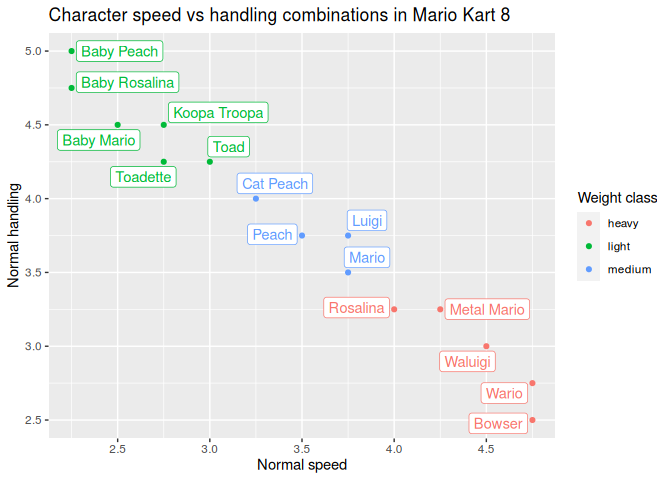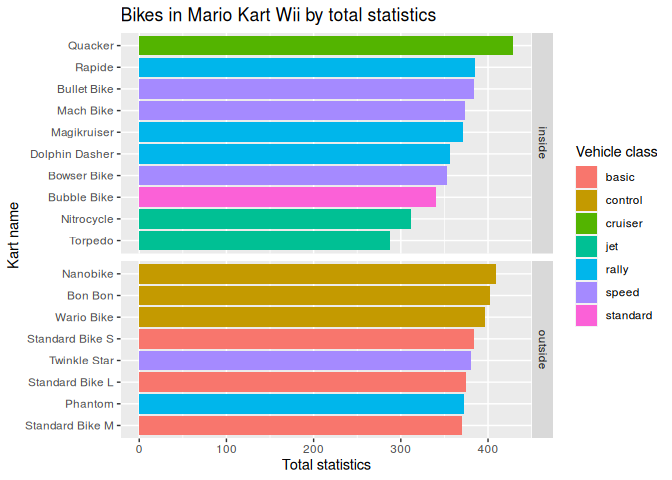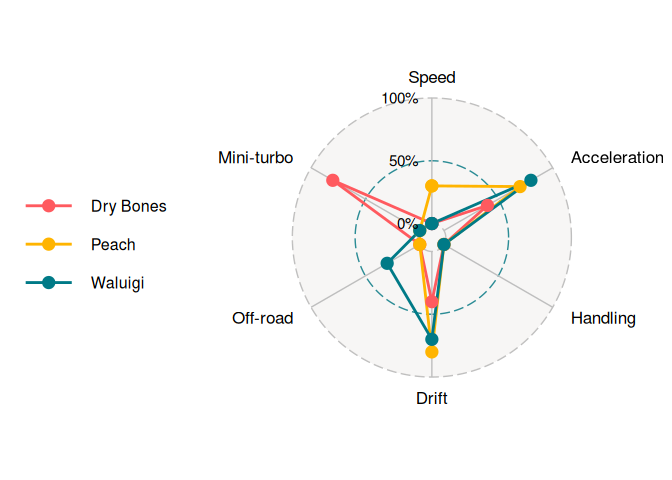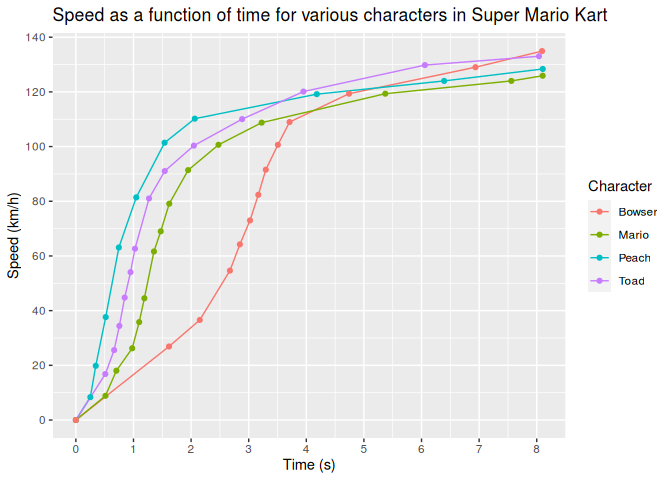## Overview

Mario Kart character and vehicle statistics datasets from different games of the series.

Currently these entries have been implemented:

• Mario Kart Wii (mkwii_characters, mkwii_vehicles)
• Mario Kart 8 (mk8_characters, mk8_vehicles, mk8_wheels, mk8_gliders)

## Installation

The development version from GitHub with:

# install.packages("devtools")
devtools::install_github("aldomann/mariokart")

## Examples

This is an example which shows how to visually compare statistics from different Mario Kart 8 characters:

library(mariokart)
library(ggplot2)
library(dplyr)
data(mkwii_characters)
data(mk8_vehicles)

mk8_characters %>%
group_by(speed_normal, handling_normal, weight_class) %>%
slice(1) %>%
ggplot() +
aes(x = speed_normal, y = handling_normal, color = weight_class, label = character) +
ggrepel::geom_label_repel(seed = 1, show.legend = FALSE) +
geom_point() +
scale_x_continuous(breaks = seq(2, 5, 0.5)) +
labs(
title = "Character speed vs handling combinations in Mario Kart 8",
x = "Normal speed",
y = "Normal handling",
color = "Weight class"
)This is an example which shows how to visually compare Mario Kart Wii karts statistics grouped by drifting type:

data(mkwii_vehicles)

mkwii_vehicles %>%
filter(vehicle_type == "bike") %>%
mutate(total_stats = rowSums(select_if(., is.numeric))) %>%
select(starts_with("vehicle"), drift_type, weight_class, total_stats) %>%
arrange(vehicle_class) %>%
ggplot() +
aes(x = reorder(vehicle, total_stats), y = total_stats, fill = vehicle_class) +
geom_col() +
labs(
title = "Bikes in Mario Kart Wii by total statistics",
x = "Kart name",
y = "Total statistics",
fill = "Vehicle class"
) +
facet_grid(drift_type ~ ., scales = "free_y", space = "free_y") +
coord_flip()This is an example which shows a visual comparison of the best chacarters per weight class in Mario Kart Wii:

data(mkwii_characters)

mkwii_characters %>%
select(-weight) %>%
mutate(total_stats = rowSums(select_if(., is.numeric))) %>%
dplyr::arrange(desc(total_stats)) %>%
group_by(weight_class) %>%
mutate_at(vars(-character, -weight_class, -total_stats), function(x) {x/10}) %>%
slice(1) %>%
ungroup() %>%
select(-weight_class, -total_stats) %>%
grid.label.size = 5,
group.point.size = 4,
group.line.width = 1,
axis.labels = c("Speed", "Acceleration", "Handling", "Drift", "Off-road", "Mini-turbo"),
axis.label.size = 4.5,
legend.text.size = 12
)A graph showing the speed as a function of time when accelerating from a standstill with various characters in Super Mario Kart:

data(smk_acceleration)

smk_acceleration %>%
filter(character %in% c("Mario", "Peach", "Toad", "Bowser")) %>%
ggplot() +
aes(x = time, y = speed, group = character, color = character) +
geom_line() +
geom_point() +
scale_x_continuous(breaks = seq(0, 8, 1)) +
scale_y_continuous(breaks = seq(0, 140, 20)) +
labs(
title = "Speed as a function of time for various characters in Super Mario Kart",
x = "Time (s)",
y = "Speed (km/h)",
color = "Character"
)